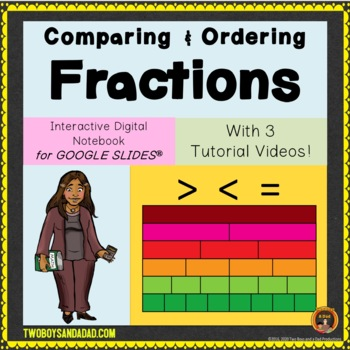DID YOU KNOW:
Seamlessly assign resources as digital activities

Learn how in 5 minutes with a tutorial resource. Try it Now

Learn More# Fractions Bundle for Comparing Fractions and Finding Equivalent Fractions3rd - 4th
Subjects
Standards
Formats Included
• Zip
•Google Apps™
Pages
90 Slides + 39 Slides + 40 Slides
\$19.00
Bundle
List Price:
\$23.75
You Save:
\$4.75
\$19.00
Bundle
List Price:
\$23.75
You Save:
\$4.75This bundle contains one or more resources with Google apps (e.g. docs, slides, etc.).

### Description

Build Fraction Number Sense with this bundle to develop comparing fractions and finding equivalent fractions. The 90 slide PowerPoint will guide students from area models, to length models to finally using number lines. Then students can practice comparing and ordering fractions and finding equivalent fractions using the Google Slides digital notebooks.

Comparing Fractions PowerPoint

The animated 6 part PowerPoint will teach students strategies for using the numerator or denominator to compare fraction sizes. They'll learn to use math tools such as area models, fraction strips (tape diagrams), and number lines. All of this builds that fraction number sense.

Comparing Fractions on Google Slides Digital Notebook

This 40 slides digital notebook uses embedded videos to make the math visual by showing students how to compare fractions using numerators and denominators. Students also practice problem-solving and reasoning about fraction sizes.

Equivalent Fractions on Google Slides Digital Notebook

This 39 slides digital notebook uses embedded videos to make the math visual by showing students how to build and identify equivalent fractions using area model, length model, and number lines. Students also practice problem-solving and reasoning about equivalent fractions.

See the individual resource for more details.

• The Comparing Fractions PowerPoint is NOT EDITABLE.
• For the Google Slides Digital Notebooks, a PDF Read Me First file will include the link to make a copy of the digital notebook to YOUR Google Drive.
• For the Digital Notebooks, the embedded videos in the digital notebook are hosted on my YouTube Channel. You and your students should have access to YouTube to play the videos directly in the notebook. If you or your students do not have access to YouTube, I have provided links to a different hosting site on which the videos can be viewed.

***************************************

♦ Be sure to click on the green ★ to follow me for updates and new product announcements!

Do you need some fresh ideas and tips? Then visit My BLOG

♦ Each time you give feedback, TPT gives you feedback credits that you use to lower the cost of your future purchases. I value your feedback greatly!

♦ If you have any questions or concerns, go ahead and contact me through the Q & A and I’ll get back to you ASAP!

********************************************************

Total Pages
90 Slides + 39 Slides + 40 Slides
Included
Teaching Duration
N/A
Report this Resource to TpT
Reported resources will be reviewed by our team. Report this resource to let us know if this resource violates TpT’s content guidelines.

### Standards

to see state-specific standards (only available in the US).
Compare two fractions with different numerators and different denominators, e.g., by creating common denominators or numerators, or by comparing to a benchmark fraction such as 1/2. Recognize that comparisons are valid only when the two fractions refer to the same whole. Record the results of comparisons with symbols >, =, or <, and justify the conclusions, e.g., by using a visual fraction model.
Explain why a fraction 𝘢/𝘣 is equivalent to a fraction (𝘯 × 𝘢)/(𝘯 × 𝘣) by using visual fraction models, with attention to how the number and size of the parts differ even though the two fractions themselves are the same size. Use this principle to recognize and generate equivalent fractions.
Compare two fractions with the same numerator or the same denominator by reasoning about their size. Recognize that comparisons are valid only when the two fractions refer to the same whole. Record the results of comparisons with the symbols >, =, or <, and justify the conclusions, e.g., by using a visual fraction model.
Recognize and generate simple equivalent fractions, (e.g., 1/2 = 2/4, 4/6 = 2/3). Explain why the fractions are equivalent, e.g., by using a visual fraction model.
Understand two fractions as equivalent (equal) if they are the same size, or the same point on a number line.